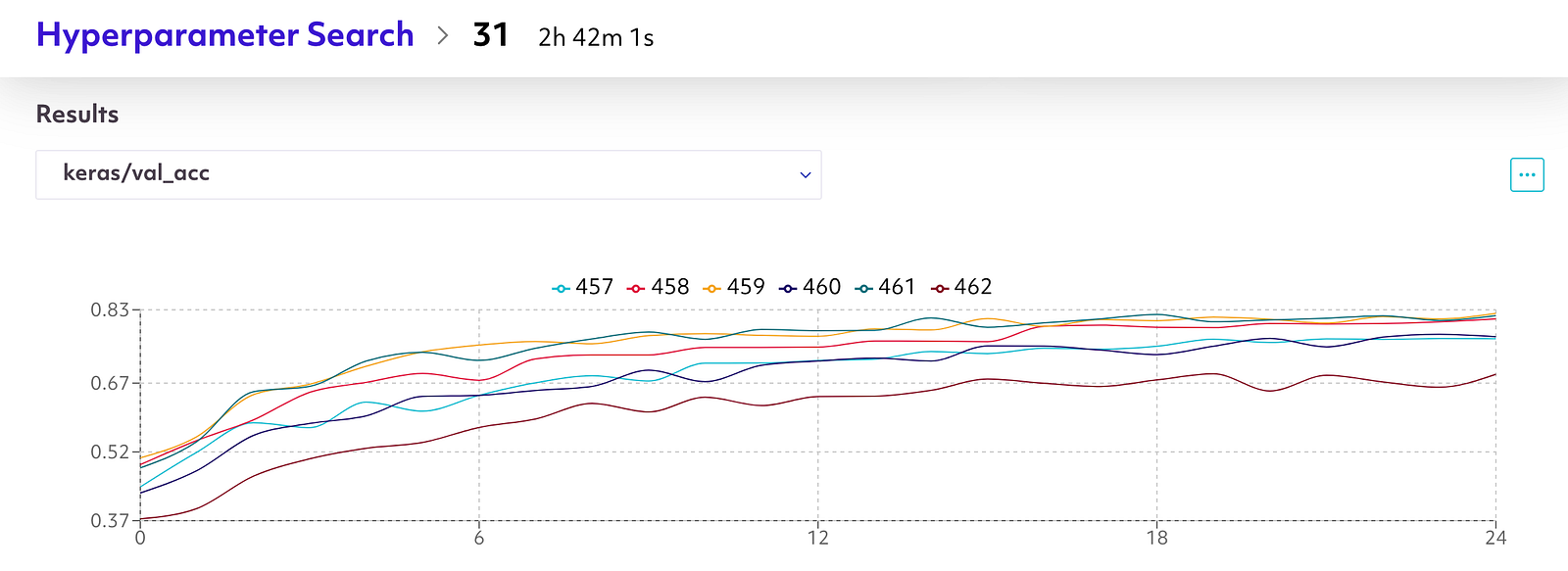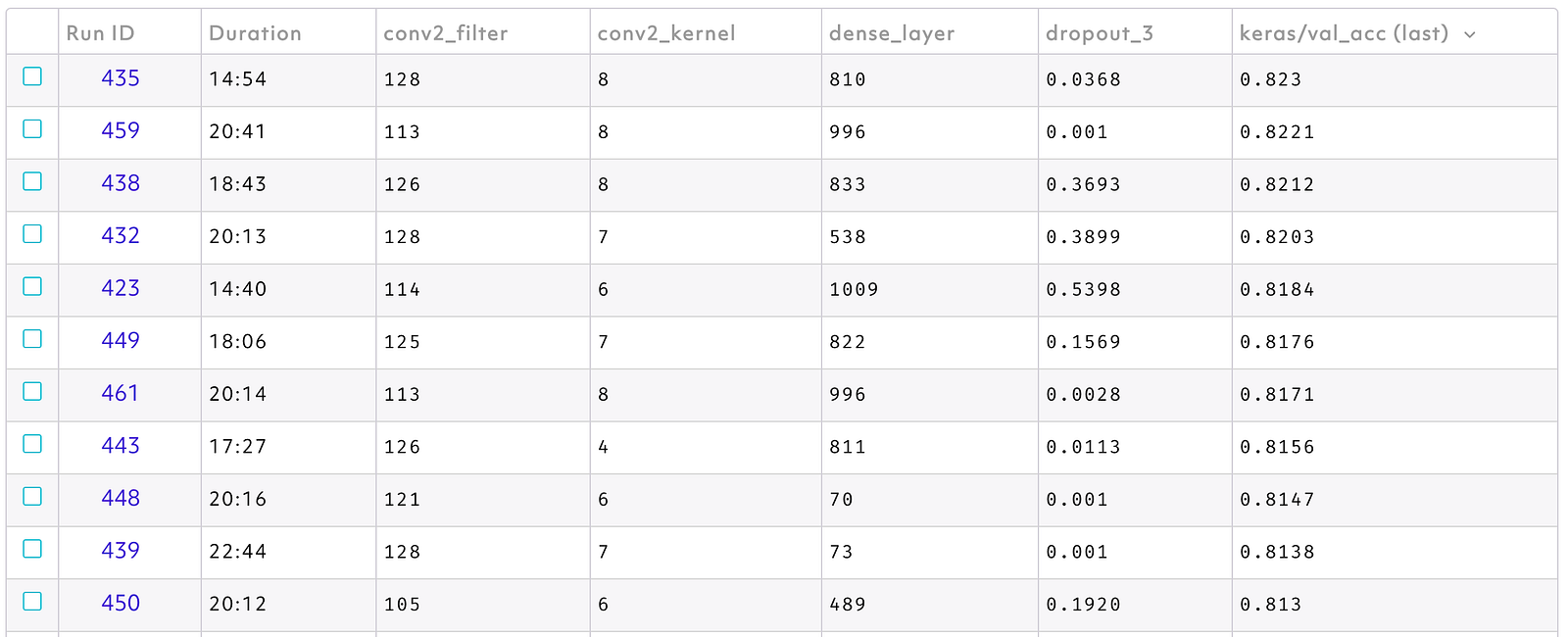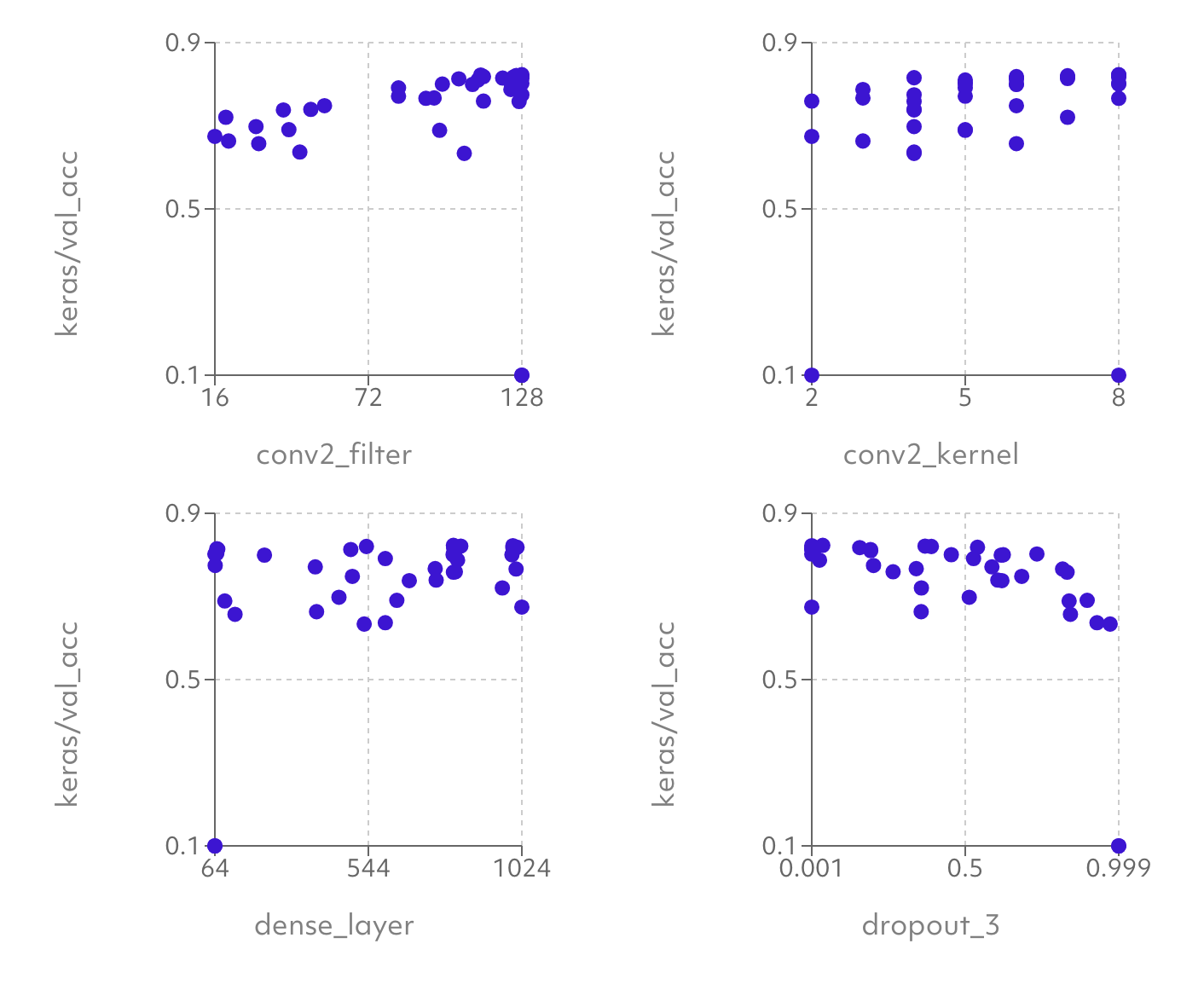Bayesian Hyperparameter Search on Spell

In addition to our existing grid and random hyperparameter strategies, Spell is excited to announce support for bayesian search. One of our awesome winter interns, Nikhil Bhatia, built a workflow that performed bayesian searches. Based on that he started working on native Spell support, which we are now launching to all users.

What is it?

Hyperparameters are any model parameters that are not optimized in the learning process, such as learning rate or the number of nodes in a specific layer of a neural network. They are notoriously hard to tune, other requiring a large amount of expensive trial and error. Two common methods involve trying a bunch of samples from the parameter space, either try a ‘grid’ (also known as a sweep) where you train the model with every combination of some preselected values for each parameter or a ‘random’ sampling from a range of potential values for each parameter.

While these techniques can be helpful to get a feel for how successful the model can be across a wide range of parameters, there is a lot of waste because after we have completed some training runs we have some knowledge about the parameter space. It makes a lot more sense to try another value near a well performing prior selection, than trying something near a poorly performing prior value. Also if there is a big area of the parameter space that hasn’t explored yet there is a lot of potential in trying a value in there rather than something near something you’ve already sampled.

One way to do a more informed search is something known as bayesian optimization. The idea here is that for a given objective function, say the accuracy of our model, we treat this as a random function and using the previously tested parameter samples and the resulting accuracy of our model after training, we create a posterior distribution over that objective function. From that we create an acquisition function which is basically our best guess of the potential a specific sample has. We then choose to test the sample which maximizes that acquisition function. There are a number of popular types of acquisition functions, our tool utilizes an upper confidence bound.

See it in action

Let’s try out this command with the CIFAR 10 dataset. This dataset contains labeled pictures of ten common objects such as cars, planes, cats, and horses. The example folder in the keras repo contains a convolutional neural network which we can use. I’ve created a version of this example that accepts some of the hyperparameters as arguments. Specifically the model is

```model = Sequential()model.add(Conv2D(32, (3, 3), padding=’same’, input_shape=x_train.shape[1:]))model.add(Activation(‘relu’))model.add(Conv2D(32, (3, 3)))model.add(Activation(‘relu’))model.add(MaxPooling2D(pool_size=(2, 2)))model.add(Dropout(0.25))
```
```model.add(Conv2D(args.conv2_filter, (args.conv2_kernel, args.conv2_kernel),padding=’same’))model.add(Activation(‘relu’))model.add(Conv2D(args.conv2_filter,(args.conv2_kernel, args.conv2_kernel)))model.add(Activation(‘relu’))model.add(MaxPooling2D(pool_size=(2, 2)))model.add(Dropout(0.25))
```
```model.add(Flatten())model.add(Dense(args.dense_layer))model.add(Activation(‘relu’))model.add(Dropout(args.dropout_3))model.add(Dense(num_classes))model.add(Activation(‘softmax’))
```

The example uses 64 for the size of 3rd and 4th convolutional layers with a kernel of (3, 3), 512 nodes for the size of the dense layer and, a final drop out of 0.5. Let’s see if we can tune those values and improve performance. After installing Spell, and cloning the examples repo `git clonehttps://github.com/spellrun/spell-examples && cd spell-examples/keras` we can run our bayesian search.

```spell hyper bayesian \-t K80 \--param conv2_filter=16:128:int \--param conv2_kernel=2:8:int \--param dense_layer=64:1024:int \--param dropout_3=0.001:0.999 \--num-runs 21 \--parallel-runs 3 \--metric keras/val_acc \--metric-agg last \-- python cifar10_cnn.py \--epochs 25 \--conv2_filter :conv2_filter: \--conv2_kernel :conv2_kernel: \--dense_layer :dense_layer: \--dropout_3 :dropout_3:
```

This will run 21 trials with up to 3 in parallel at any given time on K80 GPUs. It will be attempting to maximize the validation accuracy of the final (25th) epoch.

Results

We can take a look at the visualizations on the the Spell web console to get a picture of how these different parameters impacted performance.The validation accuracy after each of 25 epochs for the final 6 runs of this searchShows the parameters the search selected for each run as well as the resulting metric

If we head to the table and sort by `keras/val_acc` we can see that we were able to achieve an 82.3% validation accuracy with 128 filters on the convolutional layer, an 8 by 8 kernel, 810 nodes in the dense layer, and a 0.03 dropout. In general it looks like we got the best performance when we had higher number of nodes in any of the layers and a lower dropout. It was possible however to still get good performance (81.5%) with as few as 70 nodes in the dense layer. To explore these trends further we can look to the Facet charts.Facet charts with a single parameter on the x-axis and the final metric value on the y-axis

This gives a lot of interesting insights. Upping the size of the convolutional layer clearly had a significant impact. Any kernel size 4 or greater seems ok, but this is also good to know as the example model used a kernel of size 3 by 3. Interestingly, unlike the convolutional layer upping the size of the dense layer doesn’t seem as valuable. As is probably not too surprising really high dropouts didn’t perform well, but performance wasn’t particularly impacted for anything under 0.5. Since we only ran 25 epochs its not surprising that this model isn’t overfitting and a low dropout was fine. We could run another search with a much higher epoch value to tune this more finely, although from this search we can probably conclude that choosing something around a third probably won’t hamper performance too much.